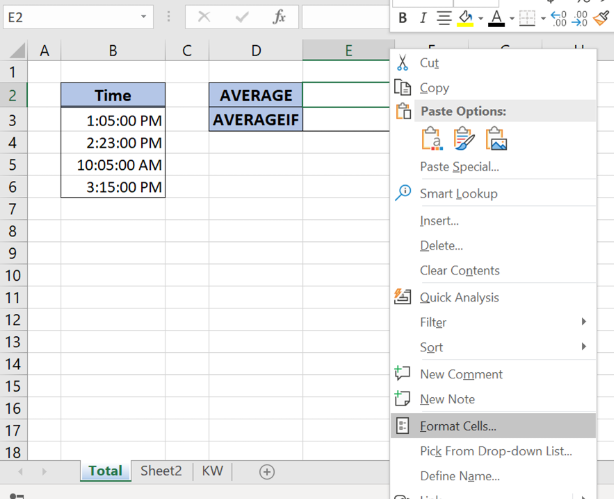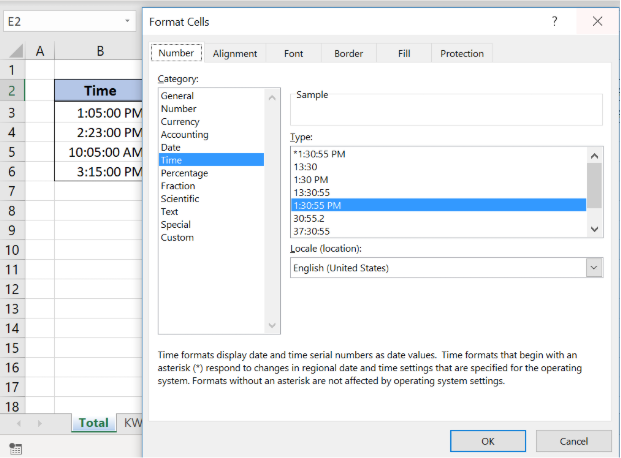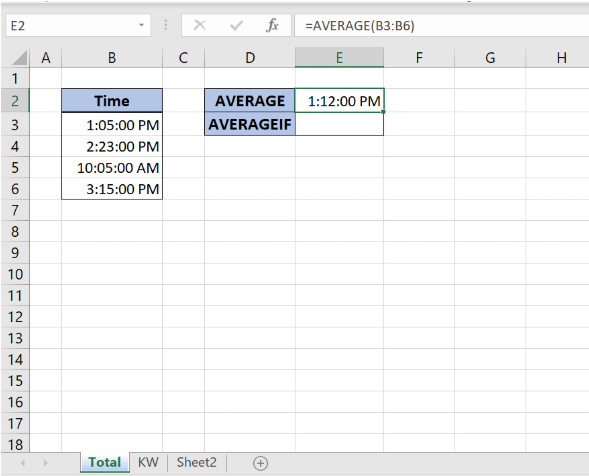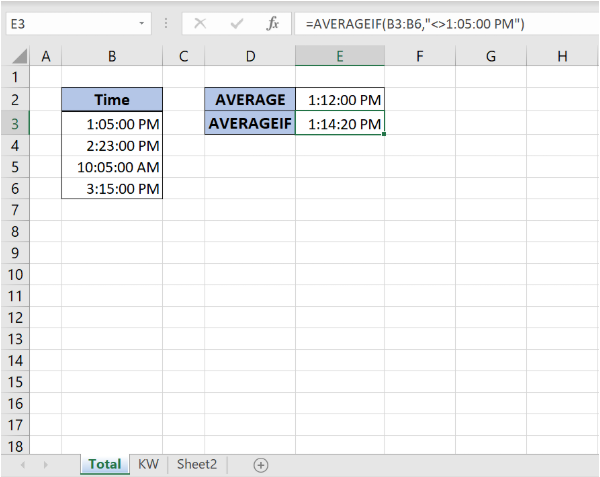Get instant live expert help with Excel or Google Sheets“My Excelchat expert helped me in less than 20 minutes, saving me what would have been 5 hours of work!”

#### Post your problem and you’ll get Expert help in seconds.

Your message must be at least 40 characters
Our professional Expert are available now. Your privacy is guaranteed.

# How to Calculate Average Time in Excel

To calculate the average time we can use the AVERAGE function. Before applying the formula in the cell we should set the Time format to display data in the time values.

This step by step tutorial will assist all levels of Excel users in learning how to get the average time.

## Calculate Average Time with AVERAGE Function

We can use the AVERAGE function to calculate the average time. Before applying the formula we should format the cell in the Time format:

• Right-click in the cell where we want to insert AVERAGE time formula
• Select Format Cells and in Number tab choose Time format
• Click OK and Time format will be set.Figure 1. Format cells to get average timeFigure 2. Set Time format to calculate average time

To get the average time we should follow the steps:

• In the cell E2 insert function` =AVERAGE(B3:B6)`

• Press Enter

The average formula has a simple syntax, we just have to select the cell range where we want to calculate the average. Since we want to get an average of times, we will select the values from column Time. As a result in the cell E2, we get an average of time from column Time.Figure 3. Calculating average time with AVERAGE function

## How to get average time with Condition

There is another average function to get the average of time with a specific condition, AVERAGEIF function:

`=AVERAGEIF(range,criteria)`

Formula syntax has two parts:

• Range, the cell range for average function

• Criteria, condition for using average function

We want to get the average of time but to exclude specific time, 1:05:00 PM. To do this we should follow several steps:

• As explained in the example above, set the Time format in the cell E3
• In the cell E3 insert the formula `=AVERAGEIF(B3:B6,"<>1:05:00 PM")`

• Press EnterFigure 4. Calculate average time with condition

As a result, we get average time that excludes specific time “<>1:05:00 PM”.

Most of the time, the problem you will need to solve will be more complex than a simple application of a formula or function. If you want to save hours of research and frustration, try our live Excelchat service! Our Excel Experts are available 24/7 to answer any Excel question you may have. We guarantee a connection within 30 seconds and a customized solution within 20 minutes.